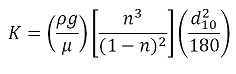## Calculator 6 - Hydraulic Conductivity - Kozeny Carmen

###The Kozeny-Carman equation is one of the most widely accepted and used derivations of permeability as a function of the characteristics of the medium.  This equation was originally proposed by Kozeny (1927) and was then modified by Carman (1937, 1956) to become the Kozeny-Carman equation.  In essence, this equation will estimate the permeability of media based on a grain size distribution. This equation is given by:Where

ρ = density

g = acceleration due to gravity

μ = dynamic viscosity

n = porosity

d10 = 10% cumulative passing (geotechnical grain size distribution)

HYDRAULIC CONDUCTIVITY ESTIMATES

Input

Groundwater temperature estimate:  oC

n (porosity):

d10 (from a geotechnical plot; % passing): m

Hydraulic Conductivity: m/s

Example:

A soil matrix has a porosity of 0.30 and a d10 value of 0.1 mm.  The groundwater is 10oC. Determine the hydraulic conductivity.

The resulting hydraulic conductivity should be 0.000023 m/s.

What would be a common error in this example?

- forgetting to convert the d10 value from mm to m.  The input should be 0.0001 m.